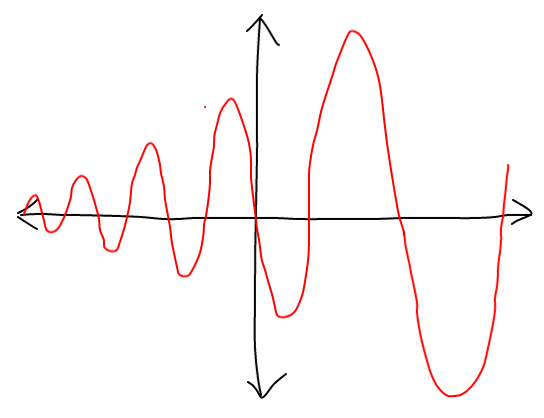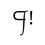# To Infinity... and Beyond!... with Waves

Can anyone give an equation of the function $f(x)$ such that

• the range of the graph is $y=(-\infty,+\infty)$?
• the graph of $f(x)$ looks like a sine wave graph?
• the period of the graph is 4?
• the graph increases in amplitude (by an arithmetic progression constant or a geometric progression constant) as $x \to +\infty$?
• the amplitude approaches $0$ as $x \to -\infty$, but there is no value of x on the graph at which the amplitude equals $0$?
• either the graph passes through the point (0,0), or...
• the x-intercept of the highest point of a crest of the graph or of the lowest point of a trough of the graph at $x=0$ is $y=1$ (if a crest is at $x=0$) or $y=-1$ (if a trough is at $x=0$)?
• the equation/graph has no transformations? (As much as possible, the equation doesn't have any unnecessary variable or number.)
• the equation contains the most "possible" amount of basic mathematical elements? (There are more basic mathematical elements than advanced ones, e.g., there are more mathematical elements related to quotients than those related to logarithms.)The picture above is merely for you to conceptualize what the graph looks like; the graph that is shown is not exactly the one I'm looking for.4 years, 5 months ago

This discussion board is a place to discuss our Daily Challenges and the math and science related to those challenges. Explanations are more than just a solution — they should explain the steps and thinking strategies that you used to obtain the solution. Comments should further the discussion of math and science.

When posting on Brilliant:

• Use the emojis to react to an explanation, whether you're congratulating a job well done , or just really confused .
• Ask specific questions about the challenge or the steps in somebody's explanation. Well-posed questions can add a lot to the discussion, but posting "I don't understand!" doesn't help anyone.
• Try to contribute something new to the discussion, whether it is an extension, generalization or other idea related to the challenge.

MarkdownAppears as
*italics* or _italics_ italics
**bold** or __bold__ bold
- bulleted- list
• bulleted
• list
1. numbered2. list
1. numbered
2. list
Note: you must add a full line of space before and after lists for them to show up correctly
paragraph 1paragraph 2

paragraph 1

paragraph 2

[example link](https://brilliant.org)example link
> This is a quote
This is a quote
    # I indented these lines
# 4 spaces, and now they show
# up as a code block.

print "hello world"
# I indented these lines
# 4 spaces, and now they show
# up as a code block.

print "hello world"
MathAppears as
Remember to wrap math in $$ ... $$ or $ ... $ to ensure proper formatting.
2 \times 3 $2 \times 3$
2^{34} $2^{34}$
a_{i-1} $a_{i-1}$
\frac{2}{3} $\frac{2}{3}$
\sqrt{2} $\sqrt{2}$
\sum_{i=1}^3 $\sum_{i=1}^3$
\sin \theta $\sin \theta$
\boxed{123} $\boxed{123}$

Sort by:

Hey,

I agree with him. I had this in class on highschool and we came to the same solution.

- 3 years, 7 months ago

Let $f : \mathbb{R} \to \mathbb{R}$ be a function with period $4$ such that the following holds : $\lim_{x \to - \infty} f(x) = 0$

Pick $x \in [0,4)$ and $k \in \mathbb{N}$. Then, $0 = \lim_{k \to \infty} f(x - 4k) = \lim_{k \to \infty} f(x) = f(x)$

Hence, $f(x) = 0 \quad \forall x \in [0,4) \\\implies f(x) = 0 \quad \forall x \in \mathbb{R}$

Evidently this function doesn't satisfy the other criteria.

We conclude that such a function doesn't exist. :P

P.S.: Based on the graph shown, I guess you had something like the following function in mind : $f(x) = e^{x} \sin \left (\frac{\pi}{2} x \right)$

- 4 years, 5 months ago

I'm sorry, but could you illustrate your proof in words? I'm bad at understanding math notations...

- 4 years, 5 months ago

Fix some value of $x$ such that $0 \leq x < 4$.

Now go 4 units back $k$ times, i.e., consider $x - 4k$. Notice that this doesn't change the value of $f$ at this point, i.e, $f(x - 4k) = f(x)$, due to periodicity of $f$.

When $k$ is very large, we, in a sense, reach $-\infty$. Hence, as the value of $f$ here is zero (intuitively), we conclude that the value of $f$ with which we began is also zero, i.e., $f(x) = 0$ where $0 \leq x < 4$.

Again, the periodicity of $f$ shows that this is the case everywhere, i.e., $f(x) = 0$ for all $x$.

- 4 years, 5 months ago